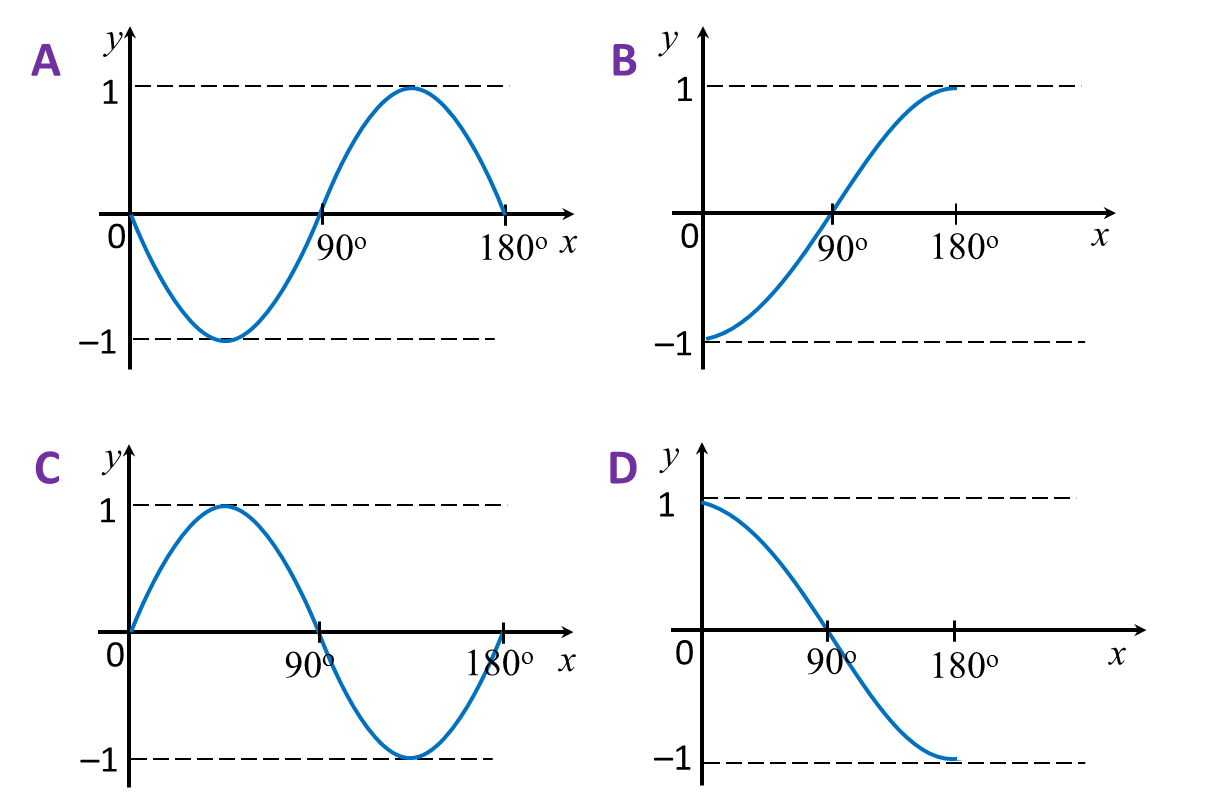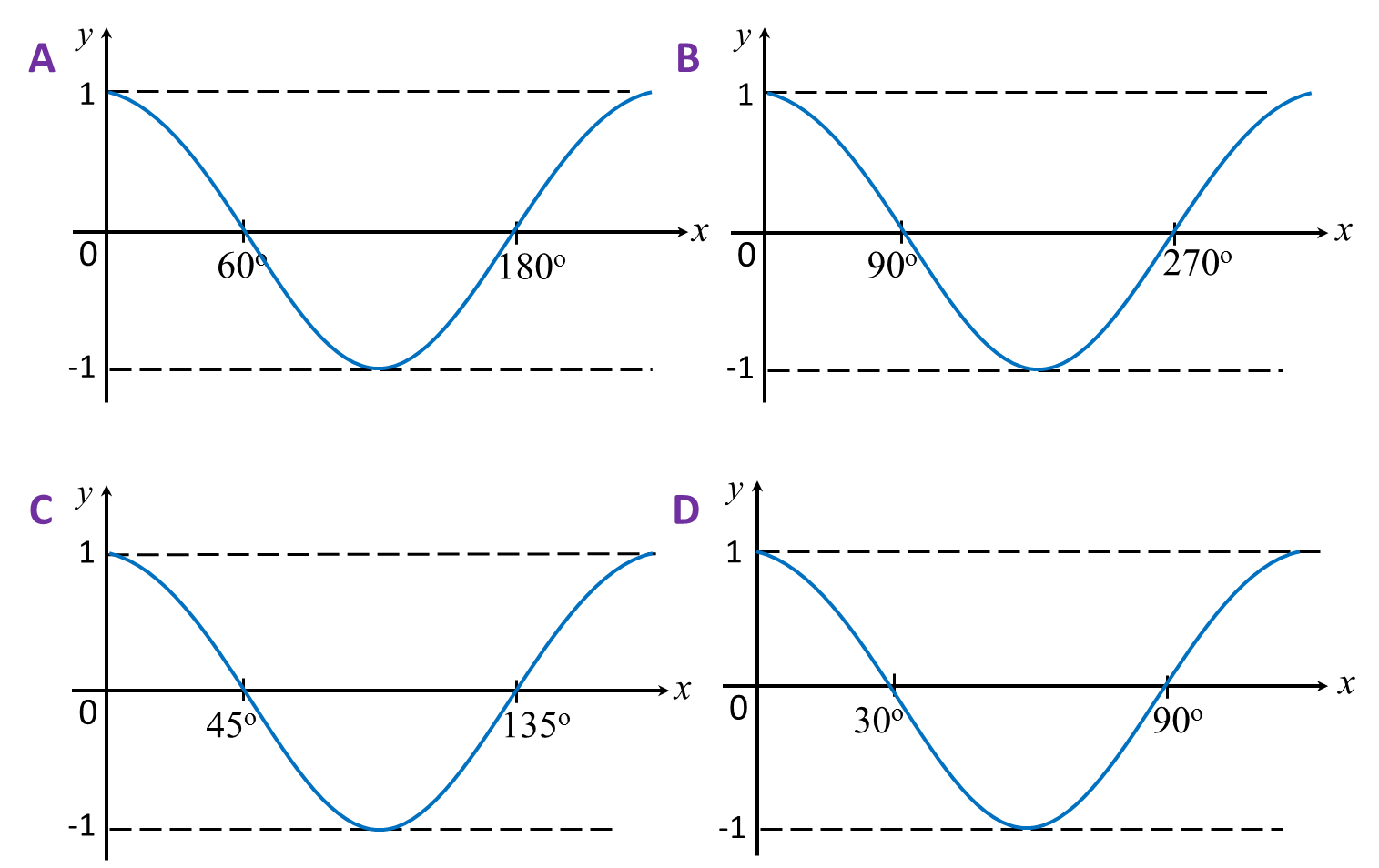# 6.3.4 Ratio and Graphs of Trigonometric Functions, SPM Paper (Short Questions)

Question 10:
Which of the following graph represents y = cos 2x?Solution:

Question 11:
Which of the following graph represents y = cos x for 0ox ≤ 180o?Solution: